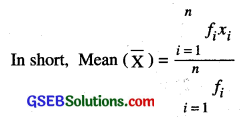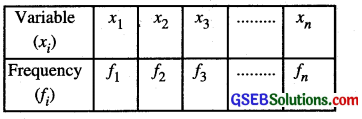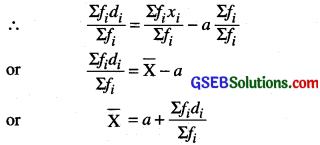# GSEB Class 10 Maths Notes Chapter 14 Statistics

This GSEB Class 10 Maths Notes Chapter 14 Statistics covers all the important topics and concepts as mentioned in the chapter.

## Statistics Class 10 GSEB Notes

We have studied the classification of given data into ungrouped as well as grouped frequency distributions. We have also learnt to represent the data pictorially in the form of various graphs such as bar graphs, histograms (including those of varying widths) and frequency polygons. In fact, we went a step further by studying certain numerical representatives of the ungrouped data, also called measures of central tendency, namely, mean, median and mode. In this chapter, we shall extend the study of these three measures i.e., mean, median and mode from ungrouped data to that of grouped data. We shall also discuss the concept of cumulative frequency, the cumulative frequency distribution and how to draw cumulative frequency curves, called ogives.

Mean of Grouped Data:
The mean (or average) of observations, is the sum of the values of all the observations divided by the total number of observations. Recall that if x1, x2, ……………, xn are observations with respective frequencies f1, f2,…. .fn, then this means observation x1 occurs f1 times, x2 occurs f2 times, and so on.

Now, the sum of the values of all the observations = f1x1 + f2x2 + + fnxn and the number of observations = f1 + f2 + ………….. + fn
So, the mean x of the data is given by
x̄ = $$\frac{f_{1} x_{1}+f_{2} x_{2}+\ldots \ldots+f_{n} x_{n}}{f_{1}+f_{2}+\ldots \ldots+f_{n}}$$Class Mark
It is assumed that the frequency of each class- interval is centred around its mid-point. So the mid piont (or class mark) of each class can be chosen to represent the observations falling in the class. The mid-poinf of a class (or its class mark) by finding the average of its upper and lower-limits. That is,

Class mark = $$\frac{\text { Upper class limit + Lower class limit }}{2}$$Mean By Assumed Mean
Consider a frequency distributionWriting the variables i.e. xi’s in ascending or descending order, then middle most (Large value) value as the assumed mean ‘a’ (say).
∴ deviation (di) = xi – a
Also, fidi =f (xi – a )
or Σfidi = Σfi (xi – a)
or Σfidi = Σ fixi – Σfia
or Σfidi = Σfixi – aΣfiStep Deviation Method or Short Cut Method
In the assumed mean method, if value of deviation i.e. d1 are divisible by a number ‘h’ (say), where h is the width of the class.
then ui = 
hui = xi – a
or xi = a + hui
or fixi = afi + hfiui
or Σfixi = Σafi + Σhfiui
or Σfixi = aΣfi + hΣfiui
or $$\frac{\Sigma f_{i} x_{i}}{\Sigma f_{i}}=a \frac{\Sigma f_{i}}{\Sigma f_{i}}+h \frac{\Sigma f_{i} u_{i}}{\Sigma f_{i}}$$
or X̄ = a + hū

Mode of grouped data
Recall from Class IX, a mode is that value among the observations which occurs most often. thus is, the value of the observation having the maximum frequency. Further. we discussed finding the mode of ungrouped data. Here, we shall discuss ways of obtaining a mode of grouped data. It is possible that more than one value may have the same maximum frequency.

In such situations, the data is said to be multimodal. Though grouped data can also be multimodal, we shall restrict ourselves to problems having a single mode only. In a grouped frequency distribution, it is not possible to determine the mode by looking at the frequencies. Here, we can only locate a class with the maximum frequency, called the modal class. The mode is a value inside the modal class. and is given by the formula:

Mode = l + $$\left(\frac{f_{1}-f_{0}}{2 f_{1}-f_{0}-f_{2}}\right)$$ × h
where l = lower limit of the modal class
h = size of the class interval (assumitig all class sizes to be equal)
f1 = frequency of the modal class,
f0 = frequency of the class preceding the modal class,
f2 = frequency of the class succeeding the modal class.

Median of Grouped Data:
The median is a measure of central tendency which gives the value of the middlemost observation in the data. Recall that for finding the median of ungrouped data, we first arrange the data values of the observations in ascending order. Then, if n is odd, the median is the $$\left(\frac{n+1}{2}\right)$$ observation.

And, if n is even, then the median will be the average of the $$\frac{n}{2}$$th and the $$\left(\frac{n}{2}+1\right)$$th observations.

In a grouped data, we may not be able to find the middle observation by looking at the cumulative frequencies as the middle observation will be some value in a class interval. It is, therefore, necessary to find the value inside a class that divides the whole distribution into two halves.

To find this class, we find the cumulative frequencies of all the classes and $$\frac{n}{2}$$. We now locate the class whose cumulative frequency is greater than (and nearest to) $$\frac{n}{2}$$. This is called the median class.

After finding the median class, we use the following formula for calculating the median.

Median = l + $$\left(\frac{\frac{n}{2}-c f}{f}\right)$$ × h
where l = lower limit of median class,
n = number of observations,
cf = cumulative frequency of class preceding the median class,
f = frequency of median class,
h = class size (assuming class size to be equal).

Which measure wotildhe best suited for a particular requirement?
The mean is the most frequently used measure of central tendency because it takes into account all the observations, and lies between the extremes, i.e., the largest and the smallest observations of the entire data. It also enables us to compare two or more distributions. For examples, by comparing the average (mean) results of students of different schools of a particular examination, we can conclude which school has a better performance.

In problems where individual observations are not important, and we wish to find out a ‘typical’ obsefvation, the median is more appropriate, e.g., finding the typical productivity rate of workers, average wage in a country, etc. These are situations where extreme values may be there. So, rather than the mean, we take the median as a better measure of central tendency.

In situations which require establishing the most frequent value or most popular item, the mode is the best choice, e.g., to find the most popular T.Y. programme being watched, the consumer item in greatest demand, the colour of the vehicle used by most of the people, etc.

Remarks:

• There is a empirical relationship between the three measures of central tendency:
3 Median = Mode + 2 Mean
• The median of grouped data with unequal class sizes can also be calculated. However, we shall not discuss it here.Graphical Representation of cumulative frequency distribution:
As we all know, pictures speak better than words. A graphical representation helps us in understanding given data at a glance. In class IX, we have represented the data through bar graphs, histograms and frequency polygons. Let us now represent a cumulative frequency distribution graphically.

To represent ‘the less than type’ graphically.
To represent the data in the table graphically, we mark the upper limits of the class intervals on the horizontal axis (x-axis) and their corresponding cumulative frequencies on the vertical axis (y-axis), choosing a convenient scale. The scale may not be the same on both the axis. Let us now plot the point corresponding to the ordered pairs given by (upper limit, corresponding cumulative frequency), on a graph paper and join them by a free hand smooth curve. The curve we get is called a cumulative frequency curve, or an ogive (of the less than type).

The term ‘ogive’ is pronounced as ‘ojeev’ and its derived from the word ogee. An ogee is a shape consisting of a concave arc flowing into a convex arc, so forming an S-shaped curve with vertical ends. In architecture, the ogee shape is one of the characteristics of the 14th and 15th century Gothic styles.

To represent ‘the more than type’ graphically, we plot the lower limits on the x-axis and the corresponding cumulative frequencies on the y- axis. Then we plot the points (lower limit, corresponding cumulative frequency), on a graph paper, and join them by a free hand smooth curve. The curve we get is a cumulative frequency curve, or an ogive, (of the more than type).